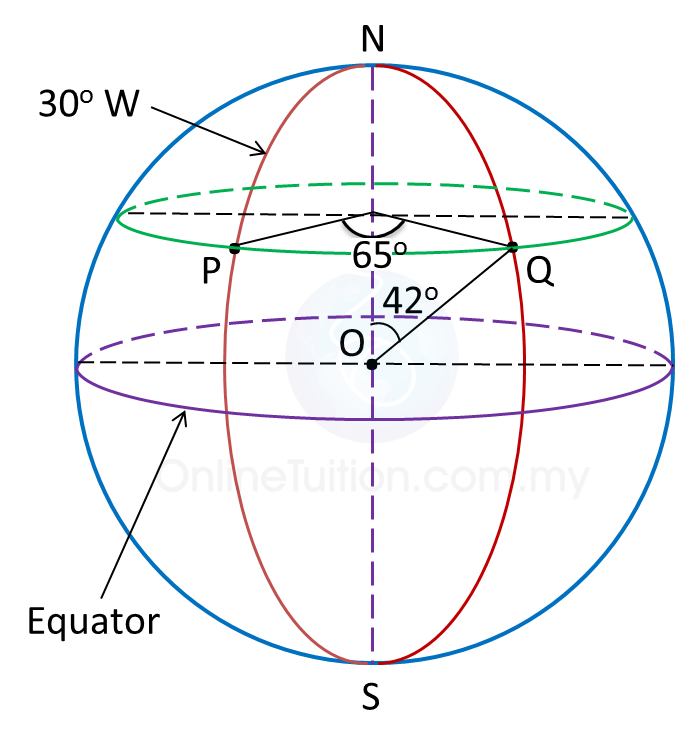# 9.5 SPM Practice (Short Questions)

Question 1
In diagram below, N is the North Pole and is the South Pole. The location of point is (40o S, 70o W) and POQ is the diameter of the earth.Find the longitude of Q.

Solution:
Since PQ is a diameter of the earth and the longitude of P is θo W, the longitude of Q is (180o – θo) E.
Longitude of = 70o W
Longitude of = (180o – 70o) E
= 110oE

Question 2
In diagram below, N is the North Pole and is the South Pole and NOS is the axis of the earth.Find the position of point Q.

Solution:
Latitude of = (90o – 42o) N
= 48o N
Longitude of = (65o – 30o) E
= 35o E
Therefore, position of Q = (48o N, 35oE).